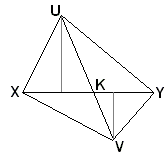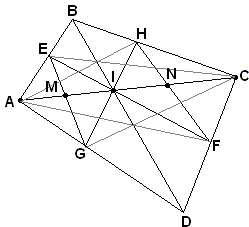## The Lepidoptera of the Quadrilateral

The Butterfly Theorem is one of the most popular and appealing in plane geometry. It has several variants and a few curious generalizations. Until very recently, all the known varieties of plane Butterflies were found in circles and their combinations. But no longer. A new variety that inhabits quadrilaterals has been just discovered. We can compare the typical species side by side:The closest to the newly discovered is that described by Steve Conrad: Through a point I on a chord AC of a circle, two other chords EF and HG are drawn. If EG and HF intersect AC in M and N, respectively, then

1/IM - 1/IA = 1/IN - 1/IC.

If we introduce a = IA, m = IM, c = IC, n = IN, then the above will appear as

 (1) 1/m - 1/a = 1/n - 1/c.

The new variety has been described by S. Kung:

### Theorem

Through the intersection I of the diagonals AC, BD of a convex quadrilateral ABCD, draw two lines EF and HG that meet the sides of ABCD in E, F, G, H. Let M and N be the intersections of EG and FH with AC. Then (1) holds.

The proof of the theorem is based on two simple lemmas.

### Lemma 1

If K is the intersection of segments XY and UV, V distinct from K, then

Area( Δ UXY)/Area( Δ VXY) = UK/VK.This is true because the two triangles share a base. Their areas therefore are in the ratio of their altitudes. The latter, along with XY and UV, cut two similar right triangles, in which the ratio of the corresponding sides is UK/VK.

### Lemma 2

Given triangles ABC and XYZ such that either ∠ABC = ∠XYZ or ∠ABC + ∠XYZ = 180°. ThenArea( Δ ABC)/Area( Δ XYZ) = AB/XY·BC/YZ.

The proof is similar to that of Lemma 1.

### Proof of TheoremThere are 12 pairs of triangles to which we may apply either Lemma 1 or Lemma 2. We get successively

 AM/IM = Area( Δ AEG)/Area( Δ IEG) IN/CN = Area( Δ IHF)/Area( Δ CHF) IC/IA = Area( Δ CBD)/Area( Δ ABD) IE/IF·IG/IH = Area( Δ IEG)/Area( Δ IHF) CH/BC·CF/CD = Area( Δ CHF)/Area( Δ CBD) AB/AE·AD/AG = Area( Δ ABD)/Area( Δ AEG) IF/IE = Area( Δ AFC)/Area( Δ AEC) IH/IG = Area( Δ AHC)/Area( Δ AGC) CD/CF = Area( Δ CAD)/Area( Δ AFC) BC/CH = Area( Δ ABC)/Area( Δ AHC) AE/AB = Area( Δ AEC)/Area( Δ ABC) AG/AD = Area( Δ AGC)/Area( Δ CAD)

Multiplying all twelve yields

AM/IM·IN/CN·IC/IA = 1,

or

(a - m)/m·n/(c - n)·c/a = 1.

Rewriting this once more we obtain

(a - m)/(am) = (c - n)/(cn),

which is the same as (1).

We should note that the new variety of butterflies admits generalizations similar to their circular relatives. For example, based on the same consideration we can claim the existence of 2N-winged butterflies dwelling on (co-diagonal) rectangles.

### References

1. S. Kung, A Butterfly Theorem for Quadrilaterals, Math Magazine 78 (Oct. 2005), pp. 314-316### Butterfly Theorem and Variants